# Complementary and Supplementary Angles Angle 1 Angle 2

• Slides: 14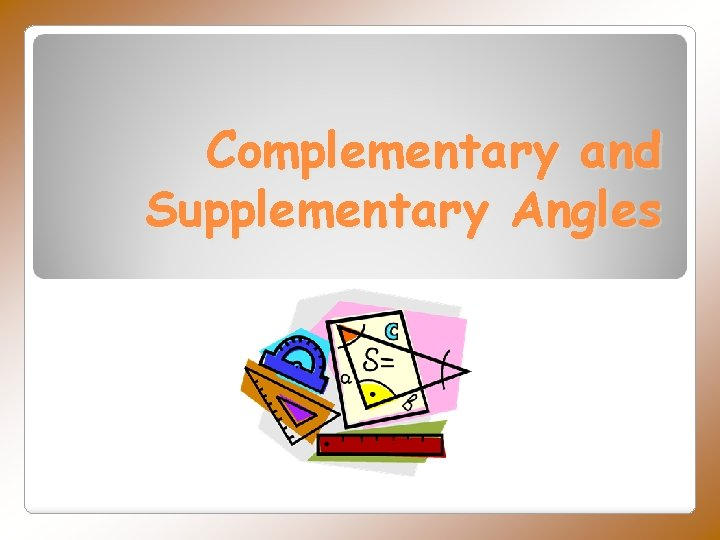Complementary and Supplementary Angles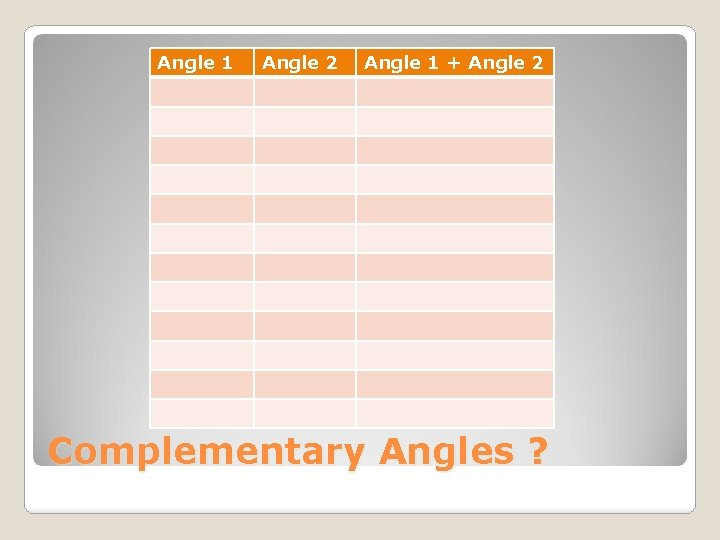Angle 1 Angle 2 Angle 1 + Angle 2 Complementary Angles ?Two angles are complementary if the sum of their angles equals 90⁰ forming a right angle. If one angle is known, its complementary angle can be found by subtracting the measure of its angle from 90⁰. Complementary AnglesWhat is the complementary angle of 43⁰? ◦ SOLUTION: 90⁰ - 43⁰ = 47⁰ ◦ So, 43⁰ and 47⁰ are complementary angles Angle A measures 25⁰ and Angle B measures 65⁰. Angle A and Angle B are complementary angles because together they create a 90⁰ angle. ◦ JUSTIFICATION: 25⁰ + 65⁰ = 90⁰ Examples of Complementary Angles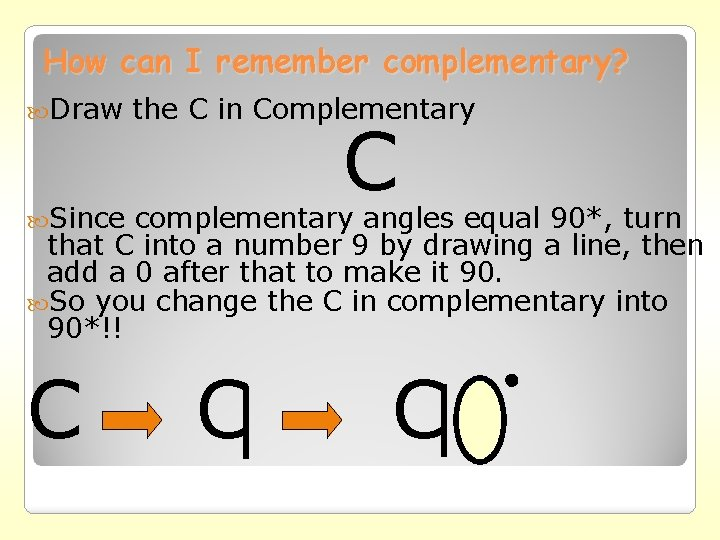How can I remember complementary? Draw the C in Complementary C Since complementary angles equal 90*, turn that C into a number 9 by drawing a line, then add a 0 after that to make it 90. So you change the C in complementary into 90*!! C C CAnother way to remember… Just remember this phrase: “It is always RIGHT to give COMPLIMENTS” A RIGHT angle is 90 ⁰ and COMPLIMENT and COMPLEMENTARY sound alike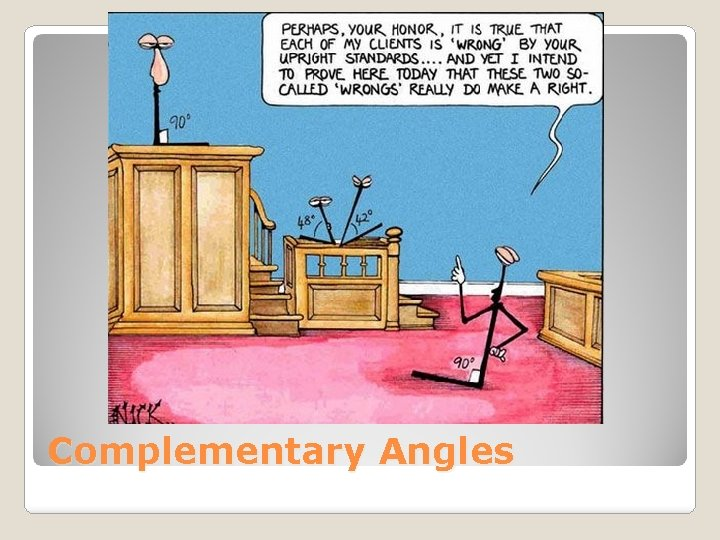Complementary AnglesClick on the link below to manipulate different angles that are Complementary. http: //www. mathopenref. com/anglecomplementar y. htmlAngle 1 Angle 2 Angle 1 + Angle 2 Supplementary Angles ?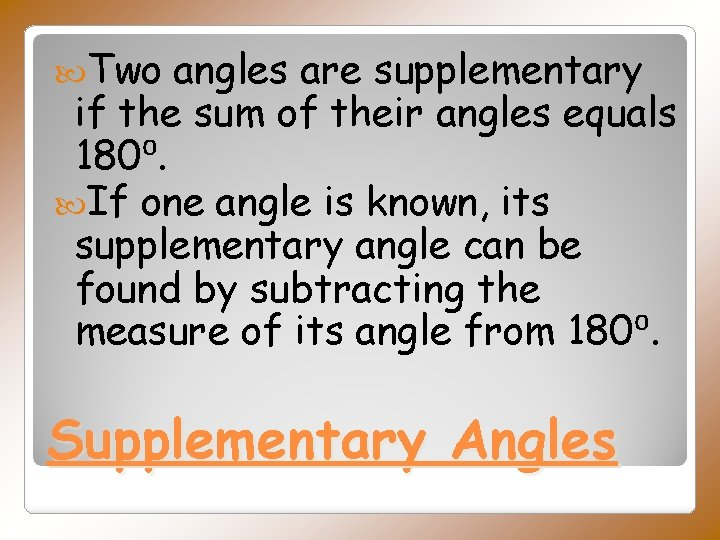Two angles are supplementary if the sum of their angles equals 180⁰. If one angle is known, its supplementary angle can be found by subtracting the measure of its angle from 180⁰. Supplementary AnglesWhat is the supplementary angle ◦ SOLUTION: 180⁰ - 143⁰ = 37⁰ of 143⁰? Angle A measures 120⁰ and Angle B measures 60⁰. Angle A and Angle B are complementary angles because together they create a 180⁰ angle. ◦ JUSTIFICATION: 120⁰ + 60⁰ = 180⁰ Example of Supplementary Angles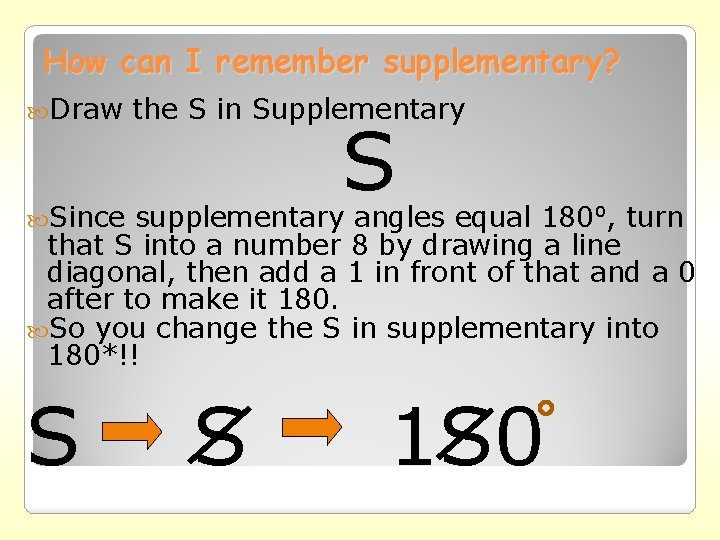How can I remember supplementary? Draw the S in Supplementary S Since supplementary angles equal 180⁰, turn that S into a number 8 by drawing a line diagonal, then add a 1 in front of that and a 0 after to make it 180. So you change the S in supplementary into 180*!! S S 1 S 0Click on the link below to manipulate different angles that are Supplementary. http: //www. mathopenref. co m/anglesupplementary. htmlComplementary Angles add up to be 90⁰ Complementary Angles look like a right angle. Supplementary Angles add up to be 180⁰ Supplementary Angles look like a straight line. Wrap it up. . .